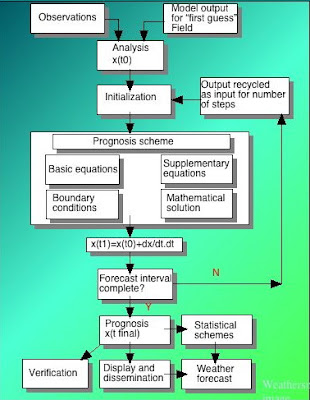## Thursday, September 3, 2009

### Predicting the Weather - How far Ahead Can We Go?

Thou, nature, art my goddess; to thy law
My services are bound.
King Lear – William Shakespeare

Computer models have been of tremendous assistance in weather prediction. But the accuracy of these models is heavily dependant on how well we can define the current state of the atmosphere, because this forms the foundations of any attempt to predict the future.

We tend to think that predicting the future using mathematics is such a complicated procedure that only scientists can understand the process but most of us have probably engaged in such predictions, perhaps without realising it at the time.

We look at a simple example that we reviewed in a previous post, but this time from a different angle. Let’s say we’re in a car moving down a freeway at 100 kph. We want to know where the car will be after 2 hours. Obviously the answer is 200 km away but let’s step back and see how be arrived with this solution.

We have, perhaps unknowingly, used a mathematical formula

D=SxT

which in words says “distance travelled equals speed multiplied by time”.

In this case S = 100 and T=2, so to obtain our answer we use the process of multiplication.

So D = 100x2, giving us our answer of 200 km.

We have therefore been able to predict the future position of our car by knowing

(1) The intitial conditions – the car is travelling at 100 kph
(2) A mathematical equation that describes the motion - D=SxT
(3) A method of “solving” the equation – in this case the process of multiplication.

Now, lets say the cruise control on our vehicle is slightly faulty and varies between 98 kph and 102 kph – where will our car now be after two hours?

Without precise knowledge of how the cruise control is varying – another equation is required for this – we don’t really know. But what we can say, using the upper and lower speeds, is that the car will be between 196 and 204 km down the road. And after 4 hours this band of uncertainty will double in size.

The same situation exists in numerical weather prediction. The current state of the atmosphere – involving the global distribution of temperature, pressure, wind humidity and other variables, both at the surface and in the upper layers of the atmosphere at a given moment form what we call the “initial conditions”, that are the starting point for all future predictions.
These are fed into the computer as an “Analysis” and are then processed via a “Prognosis scheme”, using mathematical equations. This produces a forecast, which is then fed back into the system as new initial conditions and the process is repeated until we reach the desired forecast interval.Schematic of the numerical weather prediction process

If we could produce perfect initial conditions the computer could theoretically produce perfect forecasts. But to do this we would have to know where every molecule of air is at any one moment, and what direction and speed they are all moving.

Clearly this will never be possible and this imposes an upper limit on how far ahead we can usefully forecast the weather. Just as with our car example, the errors we begin with in our initial conditions tend to magnify with time, producing a decrease in forecast accuracy the further ahead we try to go.

Another interesting feature of the atmosphere is that it appears to move through modes of “high” and “low” predictability – times where the weather patterns are persistent and predictable and others where atmospheric motion is volatile with a high degree of unpredictability.

This can be demonstrated by the use of multiple computer runs, each with slightly different initial conditions. On some occasions a small difference in these conditions will only produce a slight change in the forecasts, out to say 4 days ahead. At other times, starting with only slightly different initial conditions vastly different outcomes rapidly evolve, indicating a state of high unpredictability in the atmosphere at that time.

A simple example of predictable and unpredictable systems involves dropping a group of table tennis balls on a hollow cardboard cone. If the cone is inverted, the balls will end up in the bottom even if we drop them from slightly different positions, providing, of course, we remain within the radius of the base of the cone.

However if the cone is standing, rather than inverted, even a small difference in position from where we drop the balls will produce a large difference in outcome – that is where they come to rest. The first system is highly predictable whereas the second is not.Predictable and unpredictable systems

Weather prediction can be considered as a process in which a field of cones is negotiated, some standing and others inverted, producing areas of high and low predictability.

Taking all these factors into account it is the general consensus amongst meteorologists that meaningful weather forecasts showing real skill will eventually be achievable out to about 2 weeks ahead. After that the inherent unpredictability of the atmosphere will probably form a “glass ceiling”, impenetrable to even the most extensive observational network and the largest computers.

Reference: "Understanding Climate Change", Richard Whitaker, New Holland Publishers, 2008